Aashi Goyal — Published On June 7, 2021 and Last Modified On July 19th, 2022

This article was published as a part of the Data Science Blogathon

## Introduction

In applied Statistics and Machine Learning, Data Visualization is one of the most important skills.

Data visualization provides an important suite of tools for identifying a qualitative understanding. This can be helpful when we try to explore the dataset and extract some information to know about a dataset and can help with identifying patterns, corrupt data, outliers, and much more.

If we have a little domain knowledge, then data visualizations can be used to express and identify key relationships in plots and charts that are more helpful to yourself and stakeholders than measures of association or significance.

In this article, we will be discussing some of the basic charts or plots that you can use to better understand and visualize your data.

1. What is Data Visualization?

2. Benefits of Good Data Visualization

3. Different Types of Analysis for Data Visualization

4. Univariate Analysis Techniques for Data Visualization

• Distribution Plot
• Box and Whisker Plot
• Violin Plot

5. Bivariate Analysis Techniques for Data Visualization

• Line Plot
• Bar Plot
• Scatter Plot

## What is Data Visualization?

Data visualization is defined as a graphical representation that contains the information and the data.

By using visual elements like charts, graphs, and maps, data visualization techniques provide an accessible way to see and understand trends, outliers, and patterns in data.

In modern days we have a lot of data in our hands i.e, in the world of Big Data, data visualization tools, and technologies are crucial to analyze massive amounts of information and make data-driven decisions.

It is used in many areas such as:

• To model complex events.
• Visualize phenomenons that cannot be observed directly, such as weather patterns, medical conditions, or mathematical relationships.

## Benefits of Good Data Visualization

Since our eyes can capture the colors and patterns, therefore, we can quickly identify the red portion from blue, square from the circle, our culture is visual, including everything from art and advertisements to TV and movies.

So, Data visualization is another technique of visual art that grabs our interest and keeps our main focus on the message captured with the help of eyes.

Whenever we visualize a chart, we quickly identify the trends and outliers present in the dataset.

The basic uses of the Data Visualization technique are as follows:

• It is a powerful technique to explore the data with presentable and interpretable results.
• In the data mining process, it acts as a primary step in the pre-processing portion.
• It supports the data cleaning process by finding incorrect data and corrupted or missing values.
• It also helps to construct and select variables, which means we have to determine which variable to include and discard in the analysis.
• In the process of Data Reduction, it also plays a crucial role while combining the categories.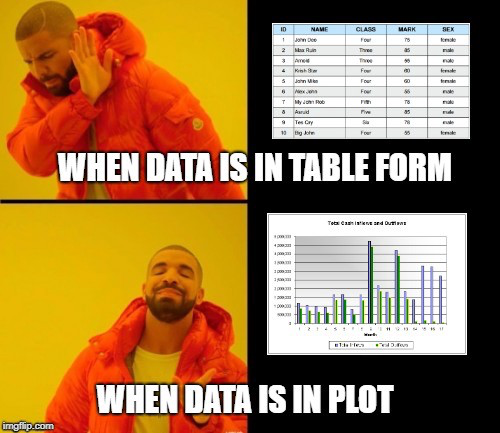## Different Types of Analysis for Data Visualization

Mainly, there are three different types of analysis for Data Visualization:

Univariate Analysis: In the univariate analysis, we will be using a single feature to analyze almost all of its properties.

Bivariate Analysis: When we compare the data between exactly 2 features then it is known as bivariate analysis.

Multivariate Analysis: In the multivariate analysis, we will be comparing more than 2 variables.

NOTE:

In this article, our main goal is to understand the following concepts:

• How do find some inferences from the data visualization techniques?
• In which condition, which technique is more useful than others?

We are not going to deep dive into the coding/implementation part of different techniques on a particular dataset but we try to find the answer to the above questions and understand only the snippet code with the help of sample plots for each of the data visualization techniques.

Now, let’s started with the different Data Visualization techniques:

## Univariate Analysis Techniques for Data Visualization

### 1. Distribution Plot

• It is one of the best univariate plots to know about the distribution of data.
• When we want to analyze the impact on the target variable(output) with respect to an independent variable(input), we use distribution plots a lot.
• This plot gives us a combination of both probability density functions(pdf) and histogram in a single plot.

Implementation:

• The distribution plot is present in the Seaborn package.

The code snippet is as follows:

Python Code:

Some conclusions inferred from the above distribution plot:

From the above distribution plot we can conclude the following observations:

• We have observed that we created a distribution plot on the feature ‘Age’(input variable) and we used different colors for the Survival status(output variable) as it is the class to be predicted.
• There is a huge overlapping area between the PDFs for different combinations.
• In this plot, the sharp block-like structures are called histograms, and the smoothed curve is known as the Probability density function(PDF).

NOTE:

The Probability density function(PDF) of a curve can help us to capture the underlying distribution of that feature which is one major takeaway from Data visualization or Exploratory Data Analysis(EDA).

### 2. Box and Whisker Plot

• This plot can be used to obtain more statistical details about the data.
• The straight lines at the maximum and minimum are also called whiskers.
• Points that lie outside the whiskers will be considered as an outlier.
• The box plot also gives us a description of the 25th, 50th,75th quartiles.
• With the help of a box plot, we can also determine the Interquartile range(IQR) where maximum details of the data will be present. Therefore, it can also give us a clear idea about the outliers in the dataset.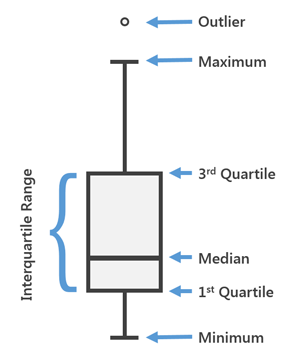Fig. General Diagram for a Box-plot

Implementation:

• Boxplot is available in the Seaborn library.
• Here x is considered as the dependent variable and y is considered as the independent variable. These box plots come under univariate analysis, which means that we are exploring data only with one variable.
• Here we are trying to check the impact of a feature named “axil_nodes” on the class named “Survival status” and not between any two independent features.

The code snippet is as follows:

`sns.boxplot(x='SurvStat',y='axil_nodes',data=hb)`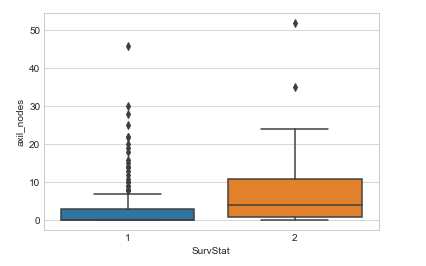Some conclusions inferred from the above box plot:

From the above box and whisker plot we can conclude the following observations:

• How much data is present in the 1st quartile and how many points are outliers etc.
• For class 1, we can see that it is very little or no data is present between the median and the 1st quartile.
• There are more outliers for class 1 in the feature named axil_nodes.

NOTE:

We can get details about outliers that will help us to well prepare the data before feeding it to a model since outliers influence a lot of Machine learning models.

### 3. Violin Plot

• The violin plots can be considered as a combination of Box plot at the middle and distribution plots(Kernel Density Estimation) on both sides of the data.
• This can give us the description of the distribution of the dataset like whether the distribution is multimodal, Skewness, etc.
• It also gives us useful information like a 95% confidence interval.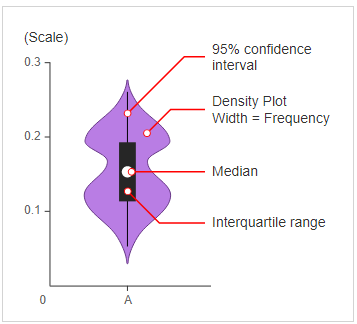Fig. General Diagram for a Violin-plot

Implementation:

• The Violin plot is present in the Seaborn package.

The code snippet is as follows:

`sns.violinplot(x='SurvStat',y='op_yr',data=hb,size=6)`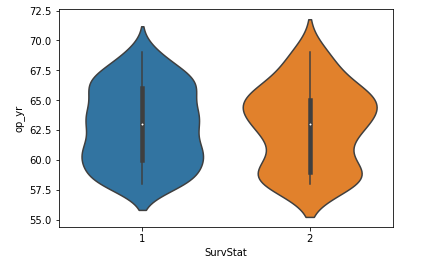Some conclusions inferred from the above violin plot:

From the above violin plot we can conclude the following observations:

• The median of both classes is close to 63.
• The maximum number of persons with class 2 has an op_yr value of 65 whereas, for persons in class1, the maximum value is around 60.
• Also, the 3rd quartile to median has a lesser number of data points than the median to the 1st quartile.

## Bivariate Analysis Techniques for Data Visualization

### 1. Line Plot

• This is the plot that you can see in the nook and corners of any sort of analysis between 2 variables.
• The line plots are nothing but the values on a series of data points will be connected with straight lines.
• The plot may seem very simple but it has more applications not only in machine learning but in many other areas.

Implementation:

• The line plot is present in the Matplotlib package.

The code snippet is as follows:

`plt.plot(x,y)`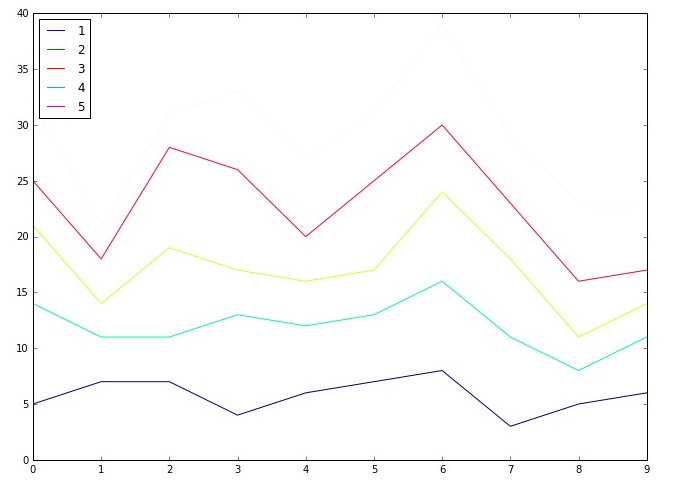Some conclusions inferred from the above line plot:

From the above line plot we can conclude the following observations:

• These are used right from performing distribution Comparison using Q-Q plots to CV tuning using the elbow method.
• Used to analyze the performance of a model using the ROC- AUC curve.

### 2. Bar Plot

• This is one of the widely used plots, that we would have seen multiple times not just in data analysis, but we use this plot also wherever there is a trend analysis in many fields.
• Though it may seem simple it is powerful in analyzing data like sales figures every week, revenue from a product, Number of visitors to a site on each day of a week, etc.

Implementation:

• The bar plot is present in the Matplotlib package.

The code snippet is as follows:

`plt.bar(x,y)`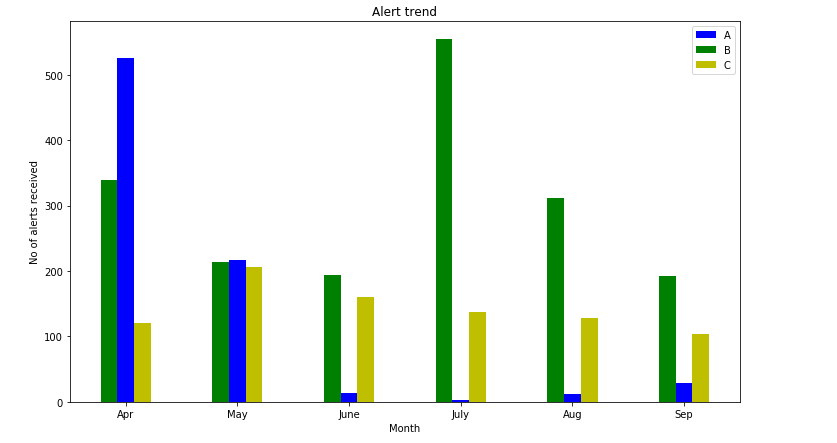Some conclusions inferred from the above bar plot:

From the above bar plot we can conclude the following observations:

• We can visualize the data in a cool plot and can convey the details straight forward to others.
• This plot may be simple and clear but it’s not much frequently used in Data science applications.

### 3. Scatter Plot

• It is one of the most commonly used plots used for visualizing simple data in Machine learning and Data Science.
• This plot describes us as a representation, where each point in the entire dataset is present with respect to any 2 to 3 features(Columns).
• Scatter plots are available in both 2-D as well as in 3-D. The 2-D scatter plot is the common one, where we will primarily try to find the patterns, clusters, and separability of the data.

Implementation:

• The scatter plot is present in the Matplotlib package.

The code snippet is as follows:

`plt.scatter(x,y)`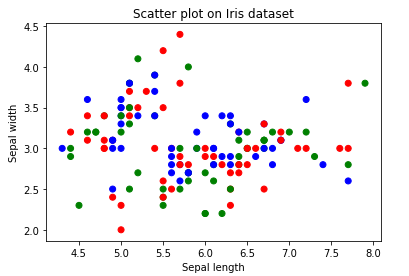Some conclusions inferred from the above Scatter plot:

From the above Scatter plot we can conclude the following observations:

• The colors are assigned to different data points based on how they were present in the dataset i.e, target column representation.
• We can color the data points as per their class label given in the dataset.

This completes today’s discussion!

## Endnotes

I hope you enjoyed the article and increased your knowledge about Data Visualization Techniques.

Please feel free to contact me on Email ([email protected])

Something not mentioned or want to share your thoughts? Feel free to comment below And I’ll get back to you.

For the remaining articles, refer to the link.

## Aashi Goyal

Currently, I am pursuing my Bachelor of Technology (B.Tech) in Electronics and Communication Engineering from Guru Jambheshwar University(GJU), Hisar. I am very enthusiastic about Statistics, Machine Learning and Deep Learning.

Your suggestions and doubts are welcomed here in the comment section. Thank you for reading my article!### Our Top Authors

•